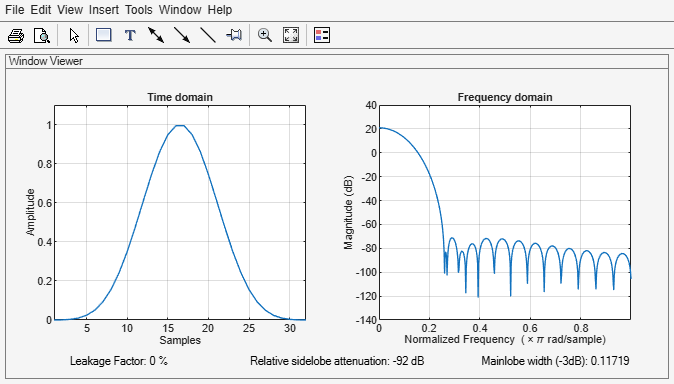blackmanharris

Minimum four-term Blackman-Harris window

Description

example

w = blackmanharris(N) returns an N-point symmetric four-term Blackman-Harris window.

w = blackmanharris(N,sflag) returns a Blackman-Harris window using the window sampling method specified by sflag.

Examples

collapse all

Create a 32-point symmetric Blackman-Harris window. Display the result using wvtool.

N = 32;
wvtool(blackmanharris(N))Input Arguments

collapse all

Window length, specified as a positive integer.

Data Types: single | double

Window sampling method, specified as:

• 'symmetric' — Use this option when using windows for filter design.

• 'periodic' — This option is useful for spectral analysis because it enables a windowed signal to have the perfect periodic extension implicit in the discrete Fourier transform. When 'periodic' is specified, the function computes a window of length L + 1 and returns the first L points.

Output Arguments

collapse all

Blackman-Harris window, returned as a column vector.

Algorithms

The equation for the symmetric four-term Blackman-Harris window of length N is

$w\left(n\right)={a}_{0}-{a}_{1}\mathrm{cos}\left(\frac{2\pi n}{N-1}\right)+{a}_{2}\mathrm{cos}\left(\frac{4\pi n}{N-1}\right)-{a}_{3}\mathrm{cos}\left(\frac{6\pi n}{N-1}\right),\text{ }0\le n\le N-1$

The equation for the periodic four-term Blackman-Harris window of length N is

$w\left(n\right)={a}_{0}-{a}_{1}\mathrm{cos}\frac{2\pi n}{N}+{a}_{2}\mathrm{cos}\frac{4\pi n}{N}-{a}_{3}\mathrm{cos}\frac{6\pi n}{N},\text{ }0\le n\le N-1$

The periodic window is N-periodic.

CoefficientValue
a00.35875
a10.48829
a20.14128
a30.01168

 harris, fredric j. “On the Use of Windows for Harmonic Analysis with the Discrete Fourier Transform.” Proceedings of the IEEE®. Vol. 66, January 1978, pp. 51–83.